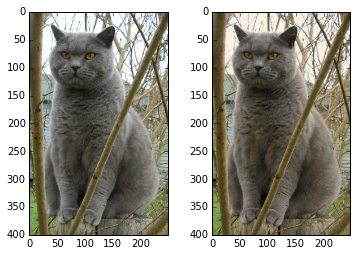# An Introduction to Matplotlib

Matplotlib is a plotting library. In this section give a brief introduction to the matplotlib.pyplot module, which provides a plotting system similar to that of MATLAB.

## Plotting

The most important function in matplotlib is plot, which allows you to plot 2D data. Here is a simple example:

Running this code produces the following plot:With just a little bit of extra work we can easily plot multiple lines at once, and add a title, legend, and axis labels:_More details about plot function in this document._

## Subplots

You can plot different things in the same figure using the subplot function. Here is an example:_More details about plot function in this document._

## Images

You can use the imshow function to show images. Here is an example: